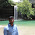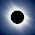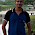# How to count Vowels and Consonants in Java String WordIn this article, we will take on a popular programming exercise of counting vowels in a word. You need to write a Java program to count how many vowels in a String, which is entered from the command prompt. It's similar to the program of counting the occurrence of characters in a String, in fact, it's a special case, where you need to count occurrences of all vowels, which includes five characters a, e, i, o and u. We will further use Scanner to get input from the user, as shown in this article. Though I have put down all code inside the main method for quick testing, if you are asked to write this program as part of your homework or during the interview, better writing a method called public int countVowels(String word) and put the logic of counting vowels there. That's a better coding style than writing everything inside the main method.

By the way, you can also use this logic to count number of consonants in a Java String. What you need to do is first count number of vowels and then subtract those characters from length of String, but remember this will only work if your String contains only alphabetic words, if it contains special character like @, _, | or numbers like 1,2,3 etc, than it will not work.

In that case you need to extend your logic to only count consonants, by extending your switch case to include rest of 21 characters from English alphabets.

## Java Program to count vowels and consonants in String

```import java.util.Scanner;

/**
* Java Program to count vowels in a String. It accept a String from command prompt
* and count how many vowels it contains. To revise, 5 letters a, e, i, o and u are
* known as vowels in English.
*/
public class VowelCounter {

public static void main(String args[]) {
System.out.println("Please enter some text");
Scanner reader = new Scanner(System.in);

String input = reader.nextLine();
char[] letters = input.toCharArray();

int count = 0;

for (char c : letters) {
switch (c) {
case 'a':
case 'e':
case 'i':
case 'o':
case 'u':
count++;
break;
default:
// no count increment
}

}
System.out.println("Number of vowels in String [" + input + "] is : " + count);
}

}

Output:
Please enter some text
How many vowels in this String
Number of vowels in String [How many vowels in this String] is : 7```

You can see that above String contains 7 vowel characters, highlighted by red font. This method is pretty quick as we are only accessing array and the using switch to compare it to another character. If you notice switch statement that you will see that we are using fall-through approach, means there is no break for all five cases, as you don't need to put break after every case. it will only increase count at one time, because increment is done at last case statement.

That's all folks on this programming exercise, It's interesting to count number of vowels or consonants in Java String, and you can do it differently as well. If you are keen to do more programming exercise, try to come out with a different solution and then compare that with this solution.

Further Learning
Java In-Depth: Become a Complete Java Engineer
Java Fundamentals: Collections
Data Structures and Algorithms: Deep Dive Using Java

Other Java Programming exercise for Practice
Java Program to print Fibonacci Series using Recursion
Write a Java program to check Armstrong number in Java
How to find if a number is palindrome in Java
How to check Prime numbers in Java
Write a Java program to calculate GCD of two numbers

1.import java.util.regex.*;

public class TotalVowelsandConsonants {

/**
* @param args
*/
public static void main(String[] args) {
// TODO Auto-generated method stub
String str = "Programming";
int vowel = 0;
int consonents = 0;
char[] charArr = str.toCharArray();
for (char c : charArr) {
if (Pattern.matches("[aeiou]", Character.toString(c)))
vowel++;
else {
consonents++;
}
}
System.out.println(vowel+" "+consonents);
}

}

2.private static void countVowelAndConsonants(String str) {
if( isEmpty(str) ) return ;

int vowelCount = 0;
int consonantsCount = 0;
String vowelStr = new String("aeiouAEIOU");

for( char ch : str.toCharArray() ) {

int unicode = (int)ch;

//First make sure given string has only alphabets, if it has chars other than alphabets skip it.

if( (unicode <= 90 && unicode >= 65 ) || (unicode <= 122 && unicode >= 97 ) ) {

//check for vowels now.
if( vowelStr.contains(ch+"") ) {
vowelCount++;
}
else {
consonantsCount++;
}

}
else {
continue;
}

}
System.out.println("vowelCount==>"+vowelCount);
System.out.println("consonantsCount==>"+consonantsCount);

}

public static boolean isEmpty(String str) {
if( null == str || "".equals(str.trim()) ) {
return true;
}
return false;

}

1.Good solution !!

2.3.#include
#include
#include
#define VOWEL_CONSONENT_PRESENT 0
#define NO_VOWEL_CONSONENT_PRESENT 1
void TestCase (int, char *);
int ConsonentVowel (int,int,char *);
int main()
{
//Test Case 1
char str1[] = "vibhay kumar";
TestCase(1, str1);
//Test Case 2
char str2[] = " ";
TestCase(2, str2);
//Test Case 3
char str3[] = "140";
TestCase(3, str3);
_getch();
}
void TestCase(int id, char *string)
{
int VowelCount = 0;
int ConsonnentCount = 0;
printf("\n\n\tTEST CASE %d", id);
int ReturnCode = ConsonentVowel(VowelCount, ConsonnentCount, string);
if (ReturnCode == 0)
{
printf("\t\nNumber of consenent count and vowel count are shown above");
}
else
{
printf("\t\nthere are neither consonent nor vowel present in string");
}
}
int ConsonentVowel(int VowelCount, int ConsonentCount, char *string)
{
while (*string)
{
if (*string == 'a' || *string == 'e' || *string == 'i' || *string == 'o' || *string == 'u')
{
VowelCount++;
}
else
{
if (*string != 0 && *string != 9 && *string !=' ' && *string !='\0')
{
ConsonentCount++;
}
}
string++;
}
if (VowelCount > 0 && ConsonentCount > 0)
{
printf("\nNumber of vowels present in string are %d", VowelCount);
printf("\nNumber of consonent present in string are %d", ConsonentCount);
return VOWEL_CONSONENT_PRESENT;
}
else
{
return NO_VOWEL_CONSONENT_PRESENT;
}
}

4.rajashekhar baligarJune 16, 2016 at 7:06 AM

int count=0;
int count1=0;
Scanner sc =new Scanner(System.in);
String in=sc.next();
for(int i=0;i<in.length();i++)
{
if(in.charAt(i)=='a'|| in.charAt(i)=='A'||in.charAt(i)=='e'||in.charAt(i)=='E'|| in.charAt(i)=='i'||in.charAt(i)=='I'||+
in.charAt(i)=='O'||in.charAt(i)=='O'||in.charAt(i)=='U'||in.charAt(i)=='u')
{
count++;
}
else
count1++;
}
System.out.println("Vowels:"+count);
System.out.println("consonants:"+count1);

5.public static void checkvowels(String str)
{
int volwel =0;
int cons = 0;
int others = 0;
String vow = "aeiouAEIOU";
for(char ch : str.toCharArray())
{
int asci_val = (int) ch;
if( (asci_val >=65 && asci_val <= 90) || (asci_val >=97 && asci_val <= 122))
{
if(vow.contains(""+ch)) {volwel++;}
else{cons++;}
}
else{others++;}
}
System.out.println(volwel + " " + cons + " " + others);
}

1.@Unknown, what is the complexity, both time and space of this solution? Can you make it better? faster?

6.How to remove duplicate counting in this program.
Any one knows?

7.@Ashesh: What do you mean duplicate counting ? Here we have to count total number so if its duplicated it will get added.

Thanks !

8.//count number of vowels and consonants in a String
private void countVowelsConsonants(String s){
String vowels = "aeiou";
int i=0;
int countVowels = 0;
int countCons = 0;
while(i<s.length()){
if(vowels.indexOf(s.charAt(i)) == -1){
countCons++;
}else{
countVowels++;
}
i++;
}
System.out.println("In the string "+s);
System.out.println("Count of vowels :"+countVowels);
System.out.println("Count of consonents :"+countCons);
}

9.public class CountVowels {
public static void main(String[] args) {
final String stringToCount = "Programming";
final String vowels = "aeiouAEIOU";
int count = 0;
for (char s : vowels.toCharArray()) {
count += stringToCount.length() - stringToCount.replaceAll(String.valueOf(s), "").length();
}
System.out.println(count);
}

}

10.package nit;
import java.io.*;

public class CountVowelDemo {

/**
* @param args
*/
public static void main(String[] args)throws Exception {
// TODO Auto-generated method stub

String str;
int vowels = 0, consonents , blanks = 0;
char ch;

System.out.print("Enter a String : ");

for(int i = 0; i < str.length(); i ++)
{
ch = str.charAt(i);

if(ch == 'a' || ch == 'A' || ch == 'e' || ch == 'E' || ch == 'i' ||
ch == 'I' || ch == 'o' || ch == 'O' || ch == 'u' || ch == 'U')
vowels ++;
else if(Character.isWhitespace(ch))
blanks ++;
}
consonents=str.length()-(vowels+blanks);
System.out.println("Vowels : " + vowels);
System.out.println("Digits : " + consonents);
System.out.println("Blanks : " + blanks);

}

}

11./*
This is very simple program to count vowels and consonants from given String even String contains any type of characters(i.e. Symbol, digit, alphabet or operator)
*/
import java.util.Scanner;

public class VowelsNConsonantsCounter {
public static void main(String args[]) {
System.out.println("Please enter some text:");
Scanner sc = new Scanner(System.in);
String input = sc.nextLine();
int count1 = 0;
int count2 = 0;

for (int i = 0; i < input.length(); i++) {
if (Character.isAlphabetic(input.charAt(i)))
switch (input.charAt(i)) {
case 'a':
case 'A':
case 'e':
case 'E':
case 'i':
case 'I':
case 'o':
case 'O':
case 'u':
case 'U':
count1++;
break;
default:
count2++;
}
}
System.out.println("Number of vowels in String [" + input + "] is : " + count1);
System.out.println("Number of Consonants in String [" + input + "] is : " + count2);
}
}